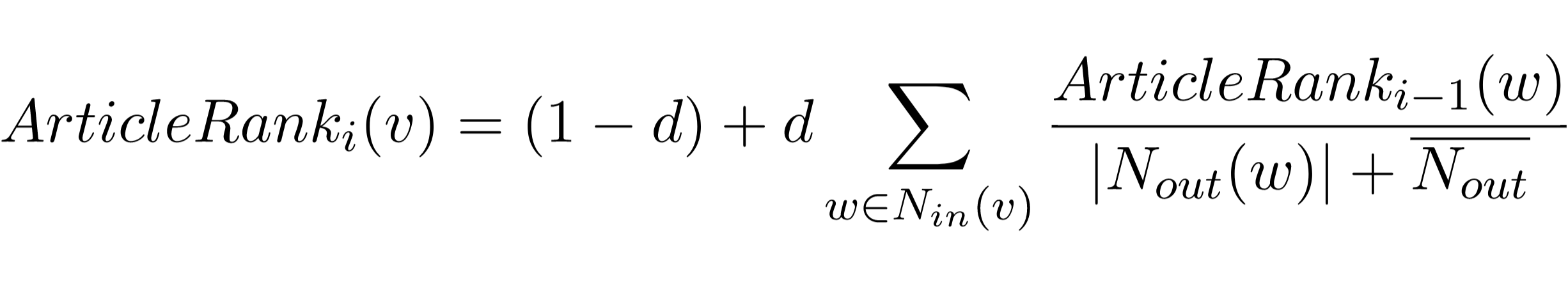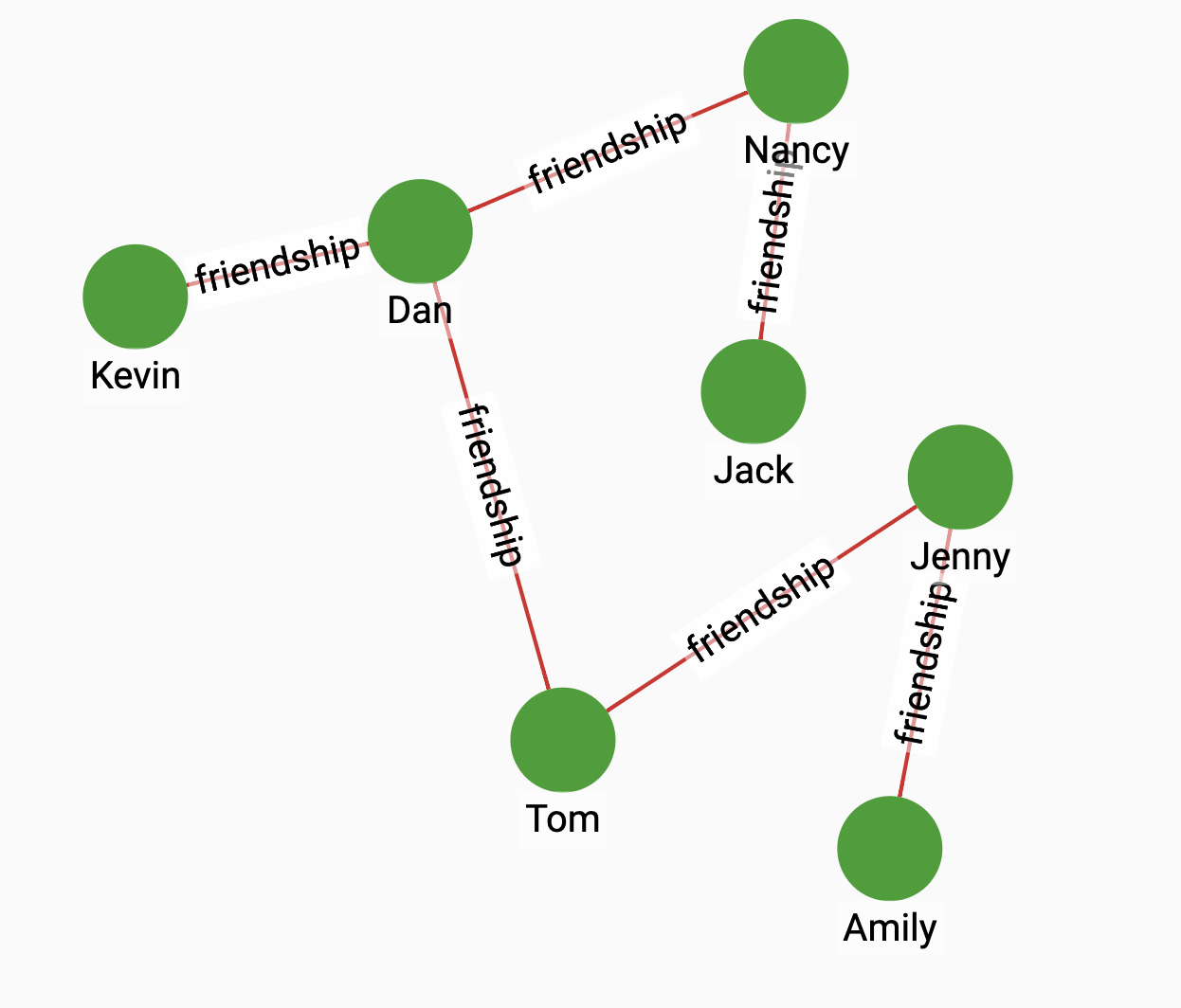# Article Rank

ArticleRank is an algorithm that has been derived from the PageRank algorithm to measure the influence of journal articles. Page Rank assumes that relationships originating from low-degree nodes have a higher influence than relationships from high-degree nodes. Article Rank modifies the formula in such a way that it retains the basic PageRank methodology but lowers the influence of low-degree nodes. The Article Rank of a node v at iteration i is defined as:Within the formula:

• $N_{in}(v)$ are the incoming neighbors and $N_{out}(v)$ are the outgoing neighbors of node v.

• d is a damping factor in [0, 1], usually set to 0.85.

• $N_{out}$ is the average outdegree.

## Specifications

``````CREATE QUERY tg_article_rank (STRING v_type, STRING e_type,
FLOAT max_change=0.001, INT max_iter=25, FLOAT damping=0.85, INT top_k = 100, BOOL print_accum = TRUE, STRING result_attr =  "", STRING file_path = "")``````

### Time complexity

The algorithm has a time complexity of $O(E*k)$, where E = number of edges, k = number of iterations. The number of iterations is data-dependent, but the user can set a maximum. Parallel processing reduces the time needed for computation.

## Parameters

Name Description Data type

`v_type`

A vertex type.

`STRING`

`e_type`

An edge type.

`STRING`

`max_change`

Article Rank will stop iterating when the largest difference between any vertex’s current score and its previous score ≤ `max_change.` That is, the scores have become very stable and are changing by less than `max_change` from one iteration to the next.

`FLOAT`

`max_iter`

Maximum number of iterations.

`INT`

`damping`

The damping factor. Usually set to 0.85.

`FLOAT`

`top_k`

The number of results with the highest scores to return.

`INT`

`print_accum`

If true, print JSON output.

`BOOL`

`result_attr`

If true, store the article rank score of each vertex in this attribute.

`STRING`

`file_path`

If true, output CSV to this file.

`STRING`

## Return value

The article rank score for each vertex.

## Example

Suppose we have the following graph:By running Article Rank on the graph, we will see that the vertex with the highest score is Dan:

• Query

• Result

``RUN QUERY tg_article_rank ("person", "friendship", _, _, _, _, _)``
``````{
"error": false,
"message": "",
"version": {
"schema": 2,
"edition": "enterprise",
"api": "v2"
},
"results": [{"@@topScores": [
{
"score": 2348294.75,
"Vertex_ID": "Dan"
},
{
"score": 1863160.625,
"Vertex_ID": "Jenny"
},
{
"score": 1442890.5,
"Vertex_ID": "Tom"
},
{
"score": 1053484.625,
"Vertex_ID": "Nancy"
},
{
"score": 739327.9375,
"Vertex_ID": "Kevin"
},
{
"score": 703562.75,
"Vertex_ID": "Amily"
},
{
"score": 498013.25,
"Vertex_ID": "Jack"
}
]}]
}``````